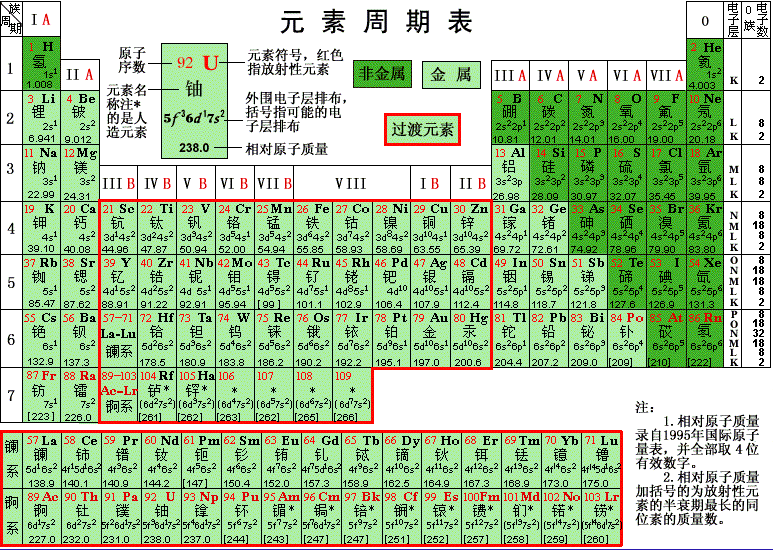# 溶液浓度的计算1/4tsp = 1.19167g
PO4 / KH2PO4 = (31+16*4)/(39+1*2+31+16*4)=95/136=0.698529
0.698529* 1.19167g = 0.832416g ＝832.416mg

832.416mg/96l水= 8.671 ppm

NO3/KNO3 = (14+16*3)/(39+14+16*3)=62/101=0.61386
10g*(5ml/500ml)＝0.1g
0.61386 * 0.1g=0.061386=61.39mg

61.39mg/40.005l=1.53ppm

## 《溶液浓度的计算》上有1条评论

1. Raphaella说道：

嘿嘿，你现在算是大师了